• 0

# JS中利用CryptoJS进行MD5/SHA256/BASE64/AES加解密的方法与示例ApiPost团队 前端 2020-06-04

CryptoJS (crypto.js) 为 JavaScript 提供了各种各样的加密算法，由于它使用起来稍微有些复杂。所以本文主要着重说一下CryptoJS进行MD5/SHA256/BASE64/AES加解密的方法与示例。

## 准备工作

ApiPost是一款功能类似Postman的工具，但是融入了文档分享功能，使用起来很方便。它内置了CryptoJS支持，所以我就以它作为调试工具了。

## MD5加密

``CryptoJS.MD5('待加密字符串').toString()``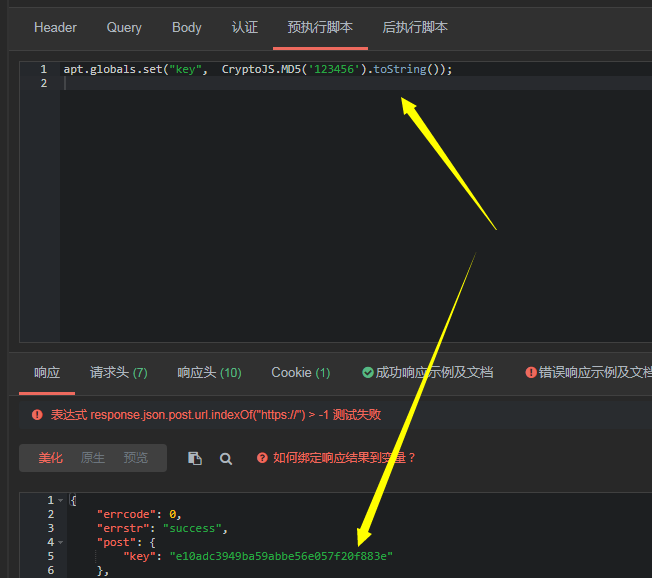## SHA256加密

``CryptoJS.SHA256('待加密字符串').toString()``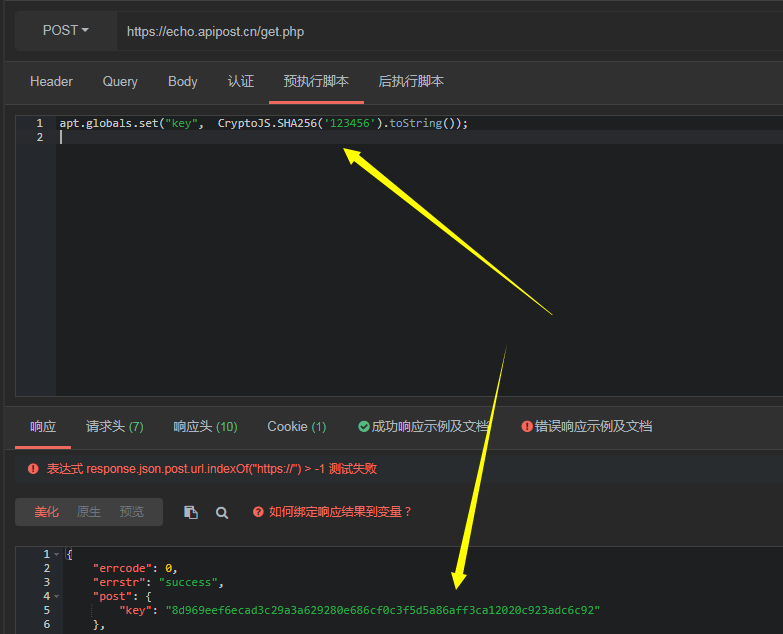## base64加密

``CryptoJS.enc.Base64.stringify(CryptoJS.enc.Utf8.parse('待加密字符串'))``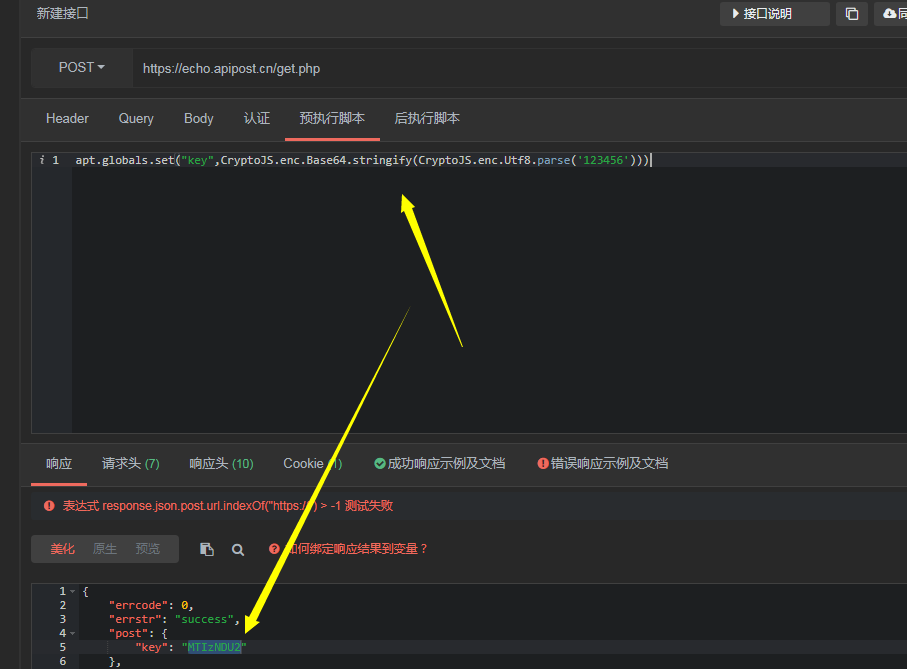## base64解密

``CryptoJS.enc.Base64.parse("待解密字符串").toString(CryptoJS.enc.Utf8)``## AES简单加密

``CryptoJS.AES.encrypt('待加密字符串', '秘钥').toString()``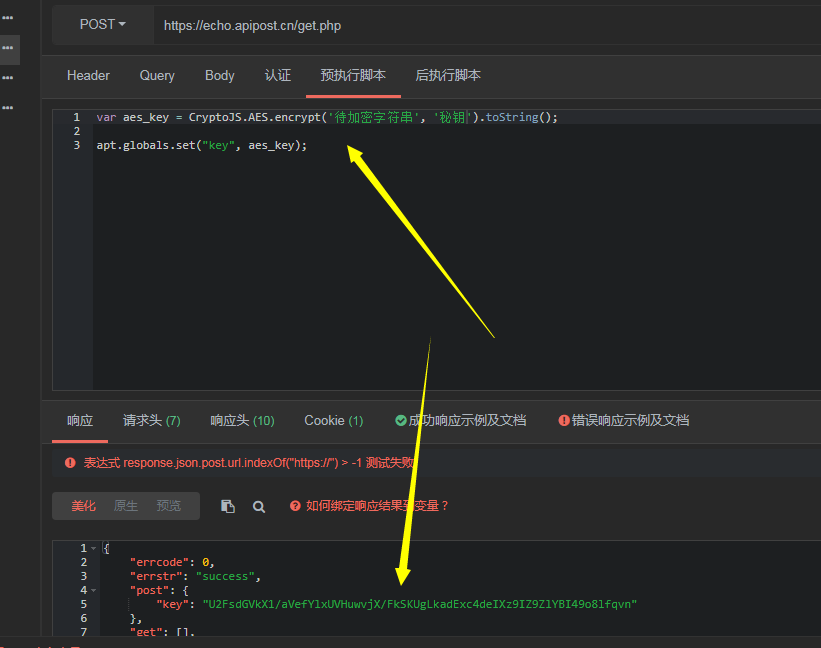## AES简单解密

``CryptoJS.AES.decrypt('待解密字符串', '秘钥').toString(CryptoJS.enc.Utf8)``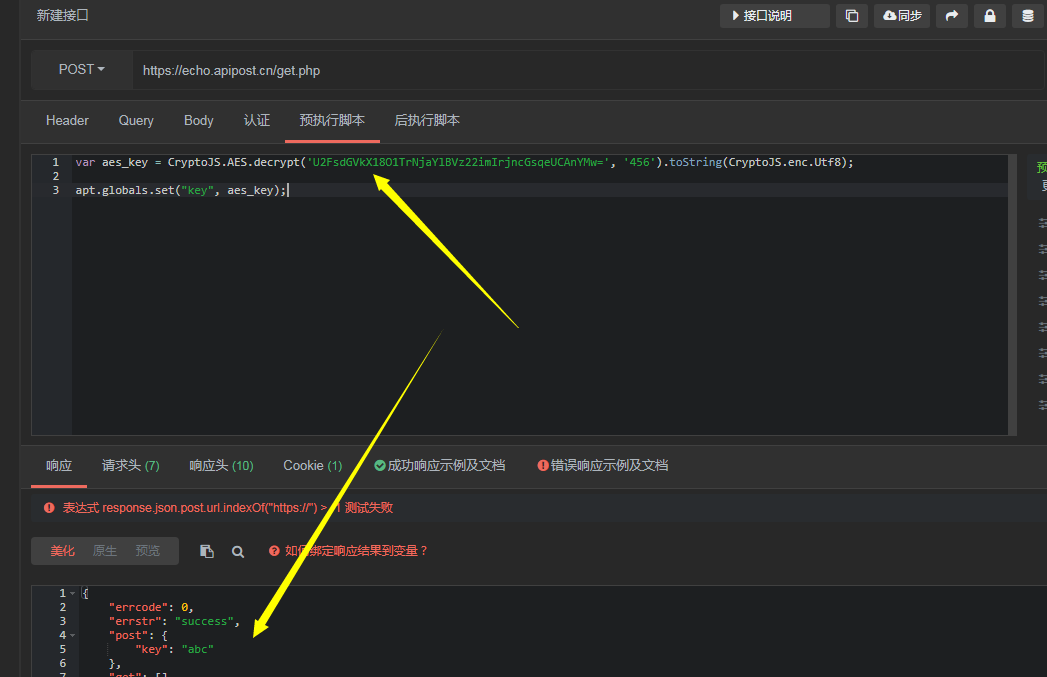## 自定义AES加解密函数

``````    const key = CryptoJS.enc.Utf8.parse("秘钥");  //十六位十六进制数作为密钥
const iv = CryptoJS.enc.Utf8.parse('偏移量');   //十六位十六进制数作为密钥偏移量

//解密方法
function Decrypt(word) {
let encryptedHexStr = CryptoJS.enc.Hex.parse(word);
let srcs = CryptoJS.enc.Base64.stringify(encryptedHexStr);
let decrypt = CryptoJS.AES.decrypt(srcs, key, { iv: iv, mode: CryptoJS.mode.CBC, padding: CryptoJS.pad.Pkcs7 });
let decryptedStr = decrypt.toString(CryptoJS.enc.Utf8);
return decryptedStr.toString();
}

//加密方法
function Encrypt(word) {
let srcs = CryptoJS.enc.Utf8.parse(word);
let encrypted = CryptoJS.AES.encrypt(srcs, key, { iv: iv, mode: CryptoJS.mode.CBC, padding: CryptoJS.pad.Pkcs7 });
return encrypted.ciphertext.toString().toUpperCase();
}

//以上方法中 mode就是加密模式，padding是填充。``````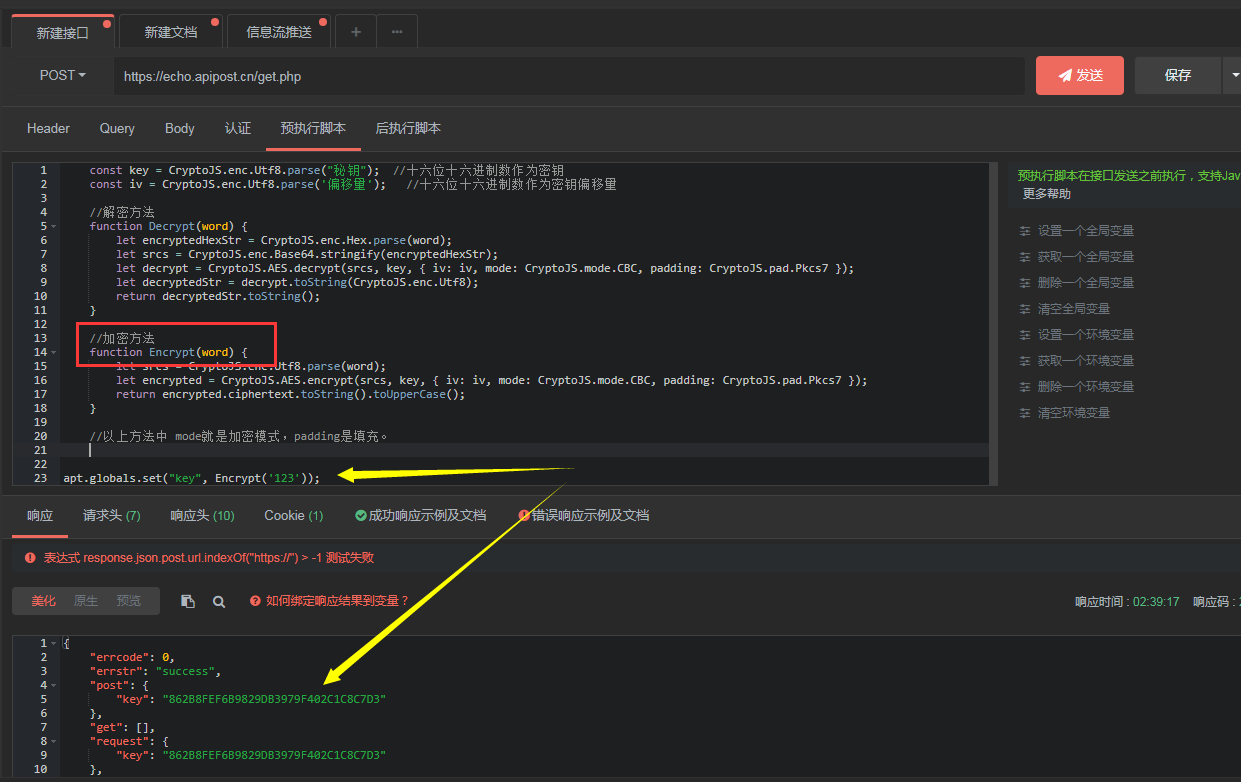## 结尾

JS的加解密类库很多，但是相对来说CryptoJS更强大一些。通过研究它的使用文档，可以发现更多惊喜。